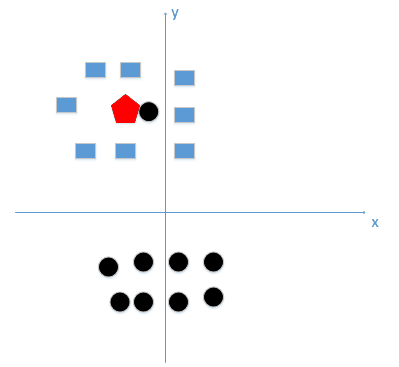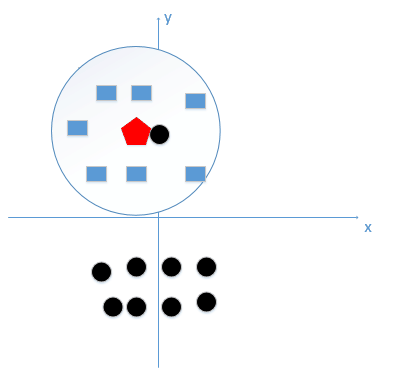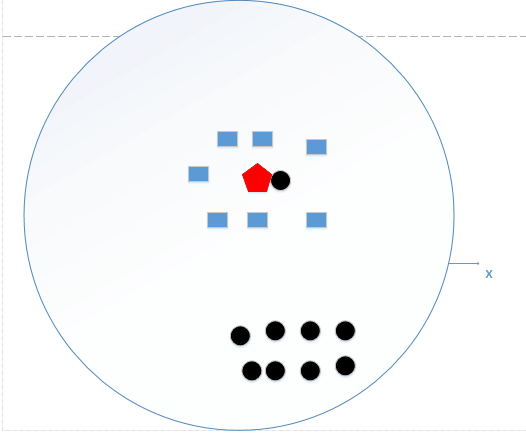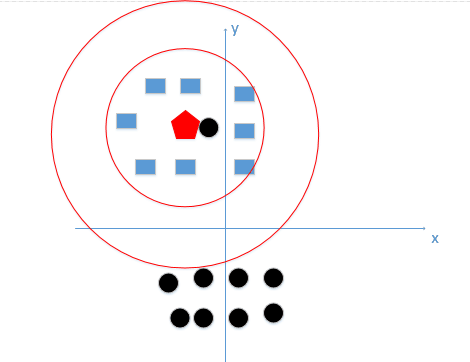# k 近邻算法的基本概念，原理

## 基本概念

k 近邻算法是一种基本分类和回归方法。本篇文章只讨论分类问题的 k 近邻法。

## 基本原理

K 近邻算法，即是给定一个训练数据集，对新的输入实例，在训练数据集中找到与该实例最邻近的 K 个实例，这 K 个实例的多数属于某个类，就把该输入实例分类到这个类中。（这就类似于现实生活中少数服从多数的思想）根据这个说法，咱们来看下引自维基百科上的一幅图：• 如果 K=3，绿色圆点的最邻近的 3 个点是 2 个红色小三角形和 1 个蓝色小正方形，少数从属于多数，基于统计的方法，判定绿色的这个待分类点属于红色的三角形一类。
• 如果 K=5，绿色圆点的最邻近的 5 个邻居是 2 个红色三角形和 3 个蓝色的正方形，还是少数从属于多数，基于统计的方法，判定绿色的这个待分类点属于蓝色的正方形一类。

# k 近邻算法中 k 的选取以及特征归一化的重要性

## 选取 k 值以及它的影响

k 近邻的 k 值我们应该怎么选取呢？## 距离的度量

$$L_{\infty}\left(x_{i}, x_{j}\right)=\max {l}\left|x{i}^{(l)}-x_{j}^{(l)}\right|$$

## 特征归一化

A [(179,42),男]     B [(178,43),男]
C [(165,36)女]      D [(177,42),男]
E [(160,35),女]

M
{j}=\max {i=1, \ldots, m} x{i j}-\min {i=1, \ldots, m} x{i j}

d\left(\left(y_{1}, \ldots, y_{n}\right),\left(z_{1}, \ldots, z_{n}\right)\right)=\sqrt{\sum_{j=1}^{n}\left(\frac{y_{j}}{M_{j}}-\frac{z_{j}}{M_{j}}\right)^{2}}


1. 1.复杂指的并不是详细或者足够大，而是这个计算模型丢失了价值，每个待分类的点遇到哪个点就分类为哪个点。
2. 2.如果考虑特性之间联系的会用到马氏距离